Area Model Multiplication - Definition with Examples

The Complete K-5 Math Learning Program Built for Your Child

• 30 Million Kids

Loved by kids and parent worldwide

• 50,000 Schools

Trusted by teachers across schools

• Comprehensive Curriculum

Aligned to Common Core

Area Model Multiplication

Area of a shape is the space occupied by the shape. The area of the given rectangle is the shaded part.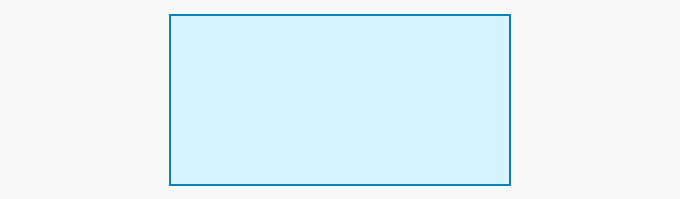If the rectangle has a length equal to 25 units and width 18 units, then this area can be calculated by finding the product 25 x 18. In other words, when you consider the product 25 x 18, geometrically we can interpret it as the area of a rectangle of length 25 units and width 18 units.

Further, we can divide this rectangle into smaller rectangles for easier calculations, and their areas can be added to get the total area of the rectangle. We can do this by breaking the lengths of the sides into smaller numbers using expanded forms. For example, 25 can be written as 20 + 5. Similarly, the width 18 can be written as 10 + 8. Using these, we can divide the original rectangle into 4 smaller rectangles as shown.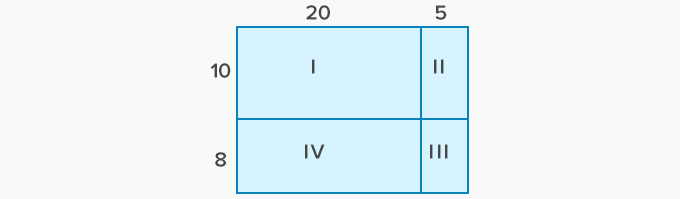Now, it is easier to calculate the areas of these smaller rectangles and their sum gives the total area or the product 25 x 18.

The rectangle I has a length of 20 units and width of 10 units making an area of 200 square units. Similarly, the rectangle II has an area equal to 10 times 5 or 50 square units. Likewise, we can calculate the areas of rectangles III and IV as 40 sq. units and 160 sq. units respectively.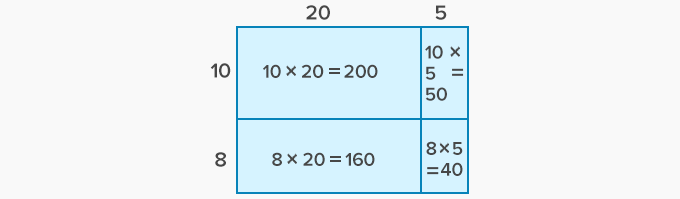Now, the total area of the rectangle of sides 25 units and 18 units is calculated by adding these partial sums.

200 + 50 + 40 + 160 = 450 sq. units

One can check using the standard algorithm to multiply two 2-digit numbers that 25 times 18 is 450.

In a rectangular auditorium, there are 36 rows of chairs with 29 chairs in each row.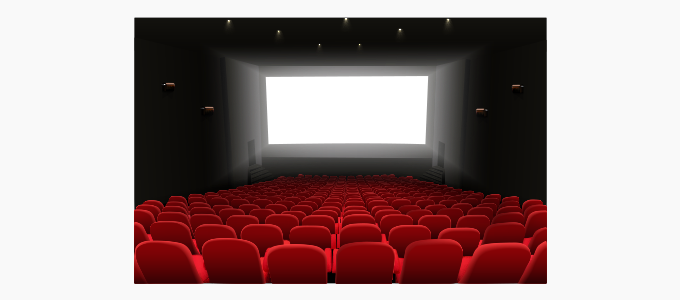To find the total number of chairs we need to find the product 36 x 29.

Step 1: Write the multiplicand and the multiplier using expanded forms.

36 = 30 + 6

29 = 20 + 9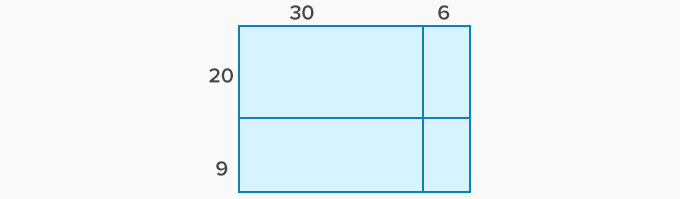Step 2: Find the areas of the smaller rectangles.Step 3: Add the partial sums to get the total area.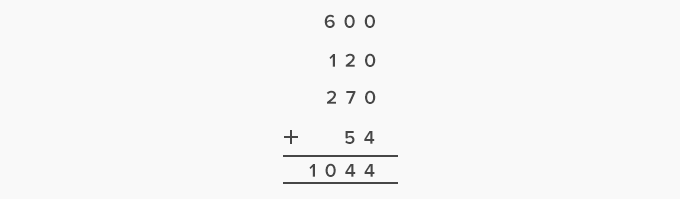Thus, there are 1044 chairs in the auditorium.

 Fun Facts Area model of multiplication uses distributive law of addition. The numbers with more than 2 digits can be multiplied by using expanded forms.

Won Numerous Awards & Honors